# ng-深度学习-课程笔记-15: 循环序列模型(Week1)

1 数学符号（Notation）

$x^{<1>}, x^{<2>}, ..., x^{<t>}, ..., x^{<q>}$ 表示一段输入序列x，比如一句文字

$y^{<1>}, y^{<2>}, ..., y^{<t>}, ..., y^{<q>}$ 表示输出序列y

$T_{x} = T_{y} = q,$ 表示长度，x和y在长度可以不相等

$x^{(i)<t>}, y^{(i)<t>}$ 表示第i个样本的第t个元素

$T_{x}^{(i)}, T_{y}^{(i)}$ 表示第i个样本的x长度和y长度

2 循环神经网络模型（Recurrent Neural Network Model）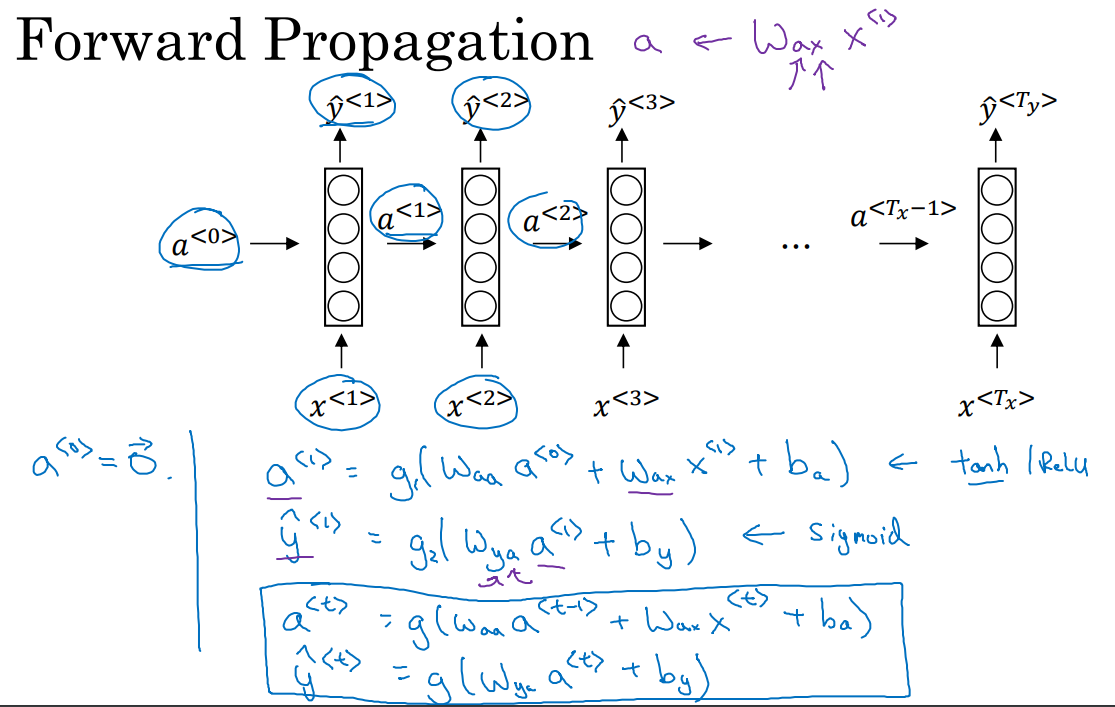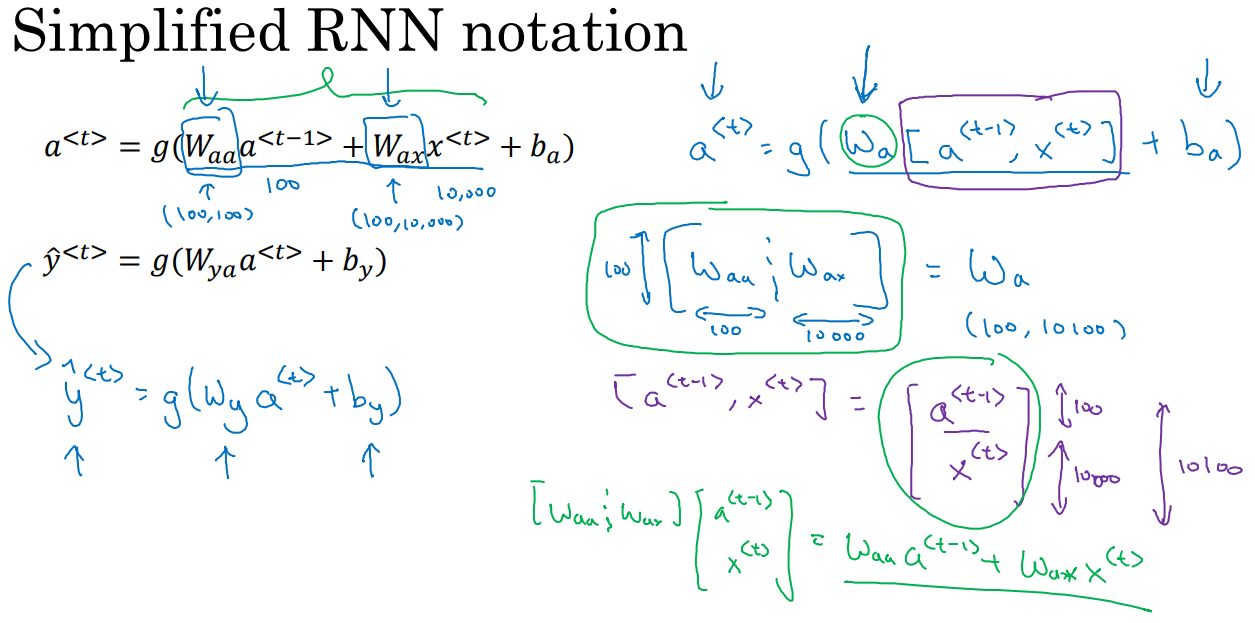3 不同类型的循环神经网络（Different types of RNNs ）

$T_{x}, T_{y}$ 不一定相同，比如下图的几个例子中，音乐生成的输入可以为长度为1或空集而输出是一段序列；感情分类的输出可以是1到5的整数而输入是一个序列；命名实体识别中，输入和输出长度相同；机器翻译中输入和输出的序列长度不同。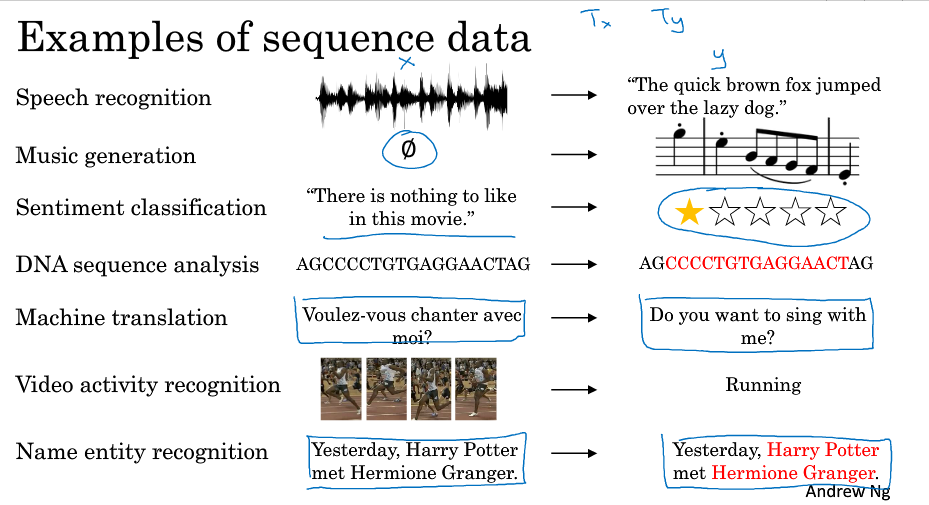One-to-one（标准的神经网络，不需要RNN）

One-to-many （比如音乐生成）

Many-to-one（比如评论的情感分类）

Many-to-many（又分为输入和输出长度相同的和不同的，比如命名实体识别和机器翻译）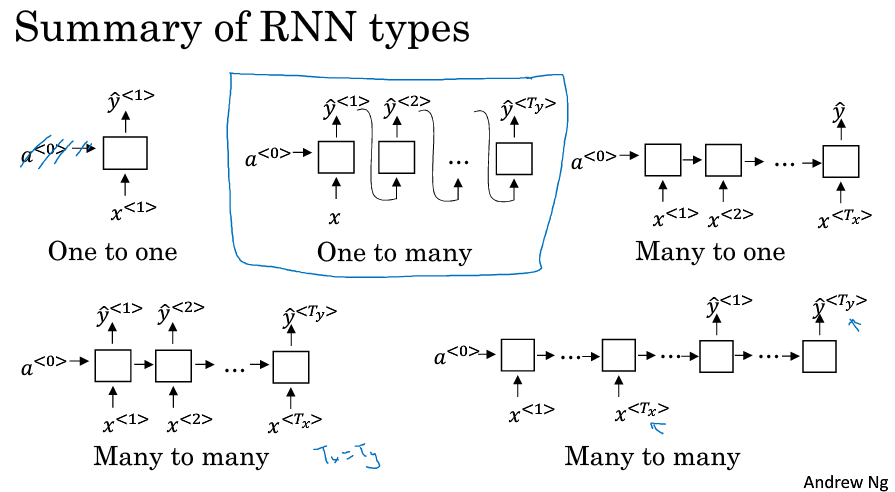4 语言模型和序列生成（Language model and sequence generation）

如何用RNN来构建一个语言模型呢？

$\hat{y}^{<1>}$ 是表示第一个位置出现的词的概率，根据softmax输出整个词典上的10002个词的概率，包括结束词和未知词，最后得到Cats的概率最大RNN和标准NN一样，随着网络层数的增加，导数有可能呈指数型减小或增加，容易发生梯度消失和梯度爆炸的问题。

6 GRU单元（Gated Recurrent Unit）

GRU，为了句子能在猫吃了很多东西之后，仍然能对be动词形式做出正确判断（was还是were），用了细胞单元来记忆信息。

GRU重要的思想是使用一个更新门 $\Gamma_u = \sigma ( W_u [ c^{<t-1>}, x^{<t>} ] + b_u )$ ，它的输出在0到1之间，大部分的输出是在非常接近0或非常接近1。

$c^{<t>} = \Gamma_u * \tilde{c}^{<t>} + (1 - \Gamma_u) * c^{<t-1>}$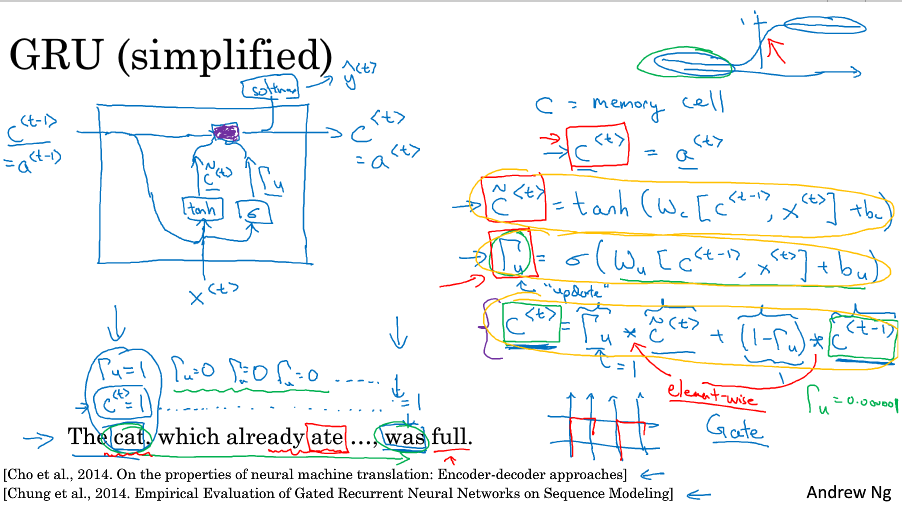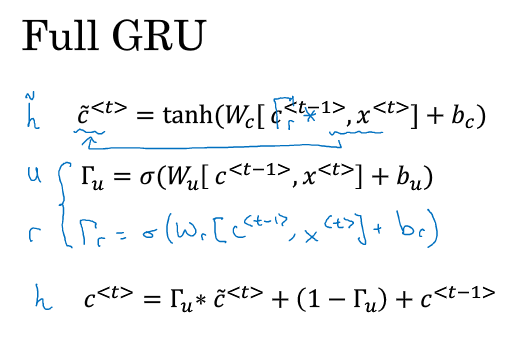7 LSTM（long short term memory ）unit

LSTM中细胞单元c不再等于激活值a，计算候选值使用的是激活值a，$\tilde{c}^{<t>} = tanh( W_c [ a^{<t-1>}, x^{<t>} ] + b_c )$

LSTM中使用三个门，更新门，遗忘门和输出门。

$\Gamma_u = \sigma ( W_u [ a^{<t-1>}, x^{<t>} ] + b_u )$

$\Gamma_f = \sigma ( W_f [ a^{<t-1>}, x^{<t>} ] + b_f )$

$\Gamma_o = \sigma ( W_o [ a^{<t-1>}, x^{<t>} ] + b_o )$

细胞的更新不再像GRU一样用单独的更新门控制，而是用更新门和遗忘门来控制

$c^{<t>} = \Gamma_u * \tilde{c}^{<t>} + \Gamma_f * c^{<t-1>}$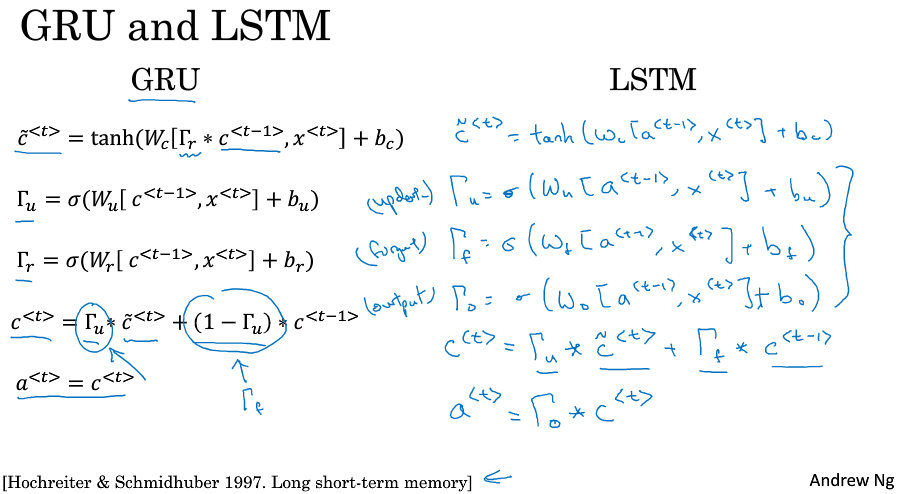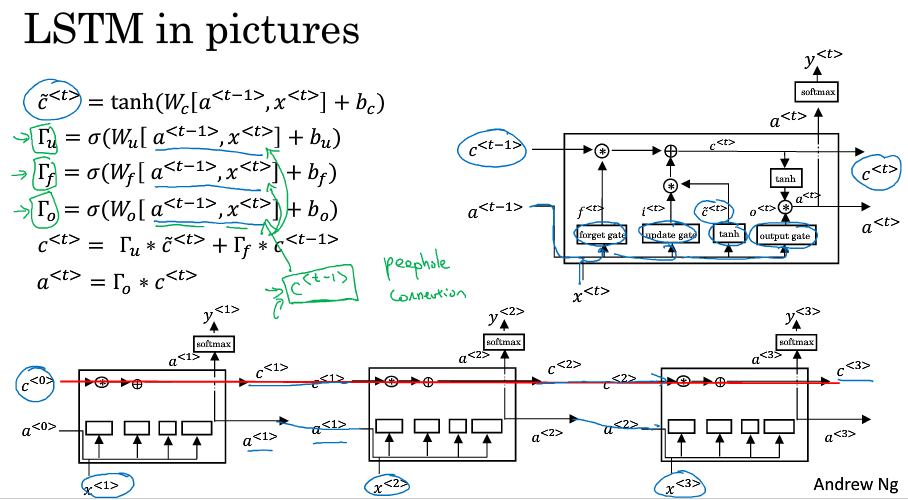GRU是在LSTM的基础上进行的简化，他的优点是更加简单，更容易创建一个更大的网络，而且它只有两个门，运行得更快。

8 双向RNN（Bidirectional RNN）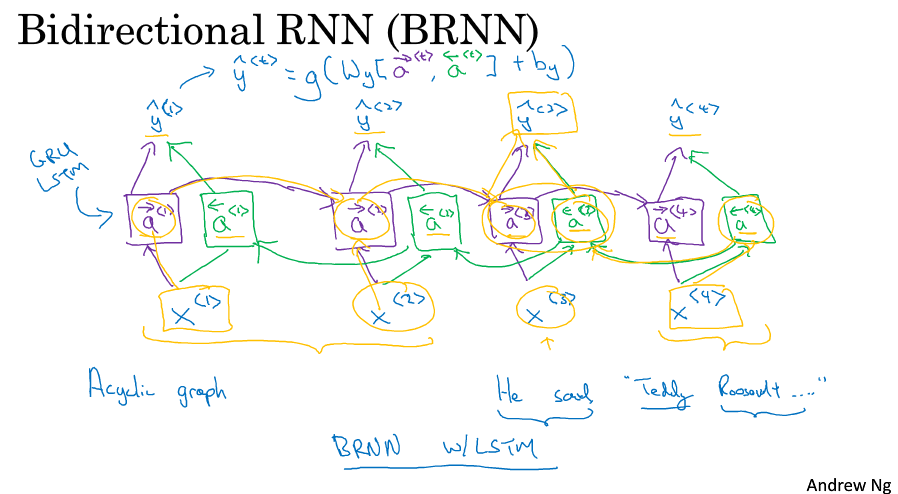9 深层循环神经网络（Deep RNNs）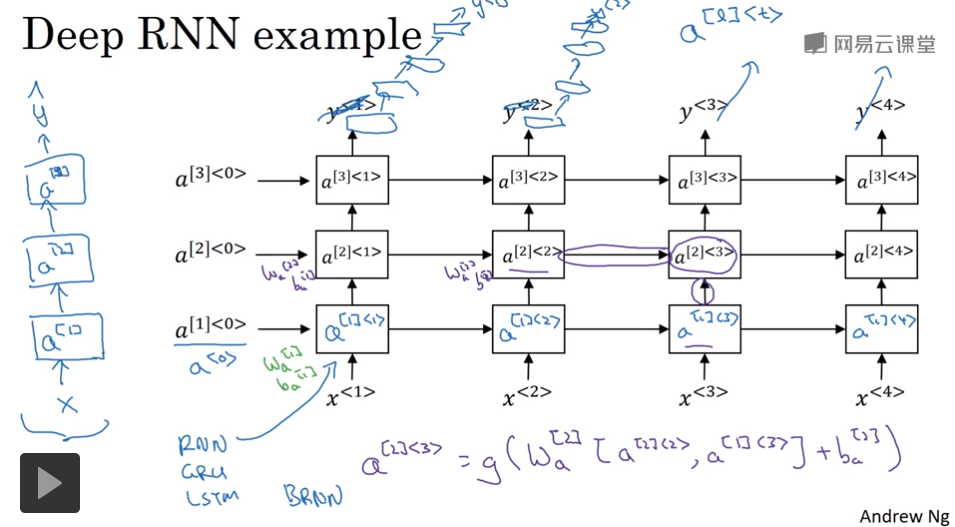posted @ 2018-06-04 21:46  PilgrimHui  阅读(426)  评论(0编辑  收藏  举报